Technical Article

# Negative Feedback, Part 7: Frequency-Dependent Feedback

November 29, 2015 by Robert Keim

Learn why the frequency response of a feedback network can seriously, and sometimes unexpectedly, degrade stability.

### Supporting Information

Just so you don’t have to switch pages if you want to ponder the general feedback structure, here is the diagram presented in the first article: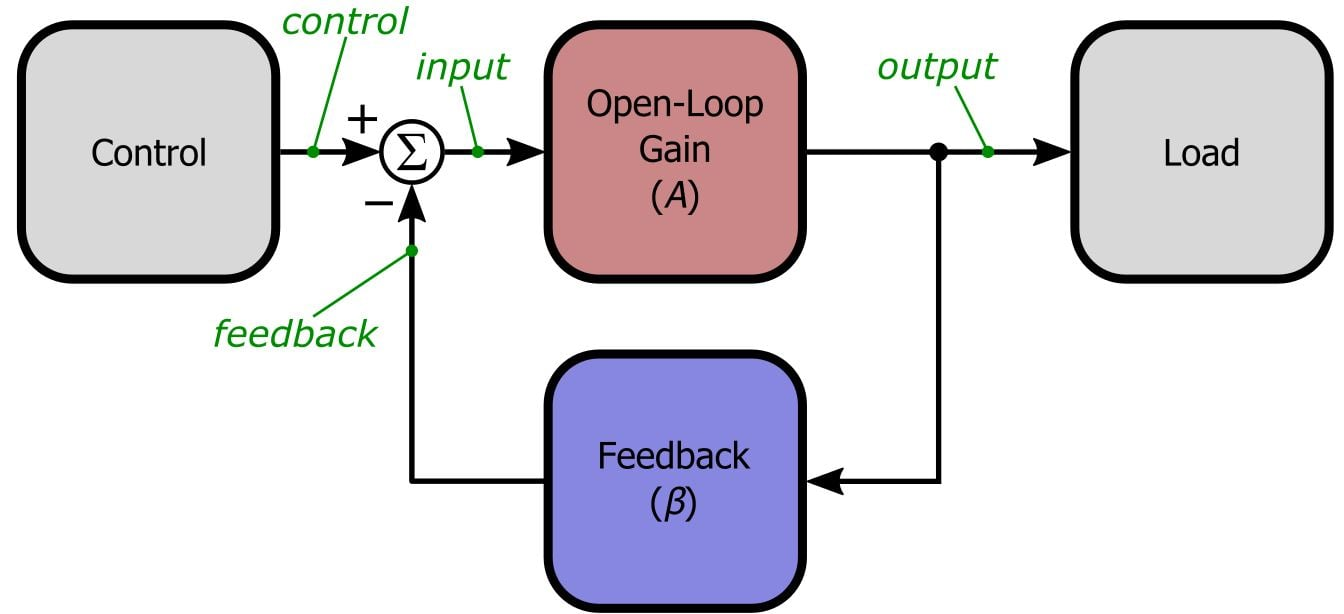And here is the LTSpice simulation circuit introduced in Part 5: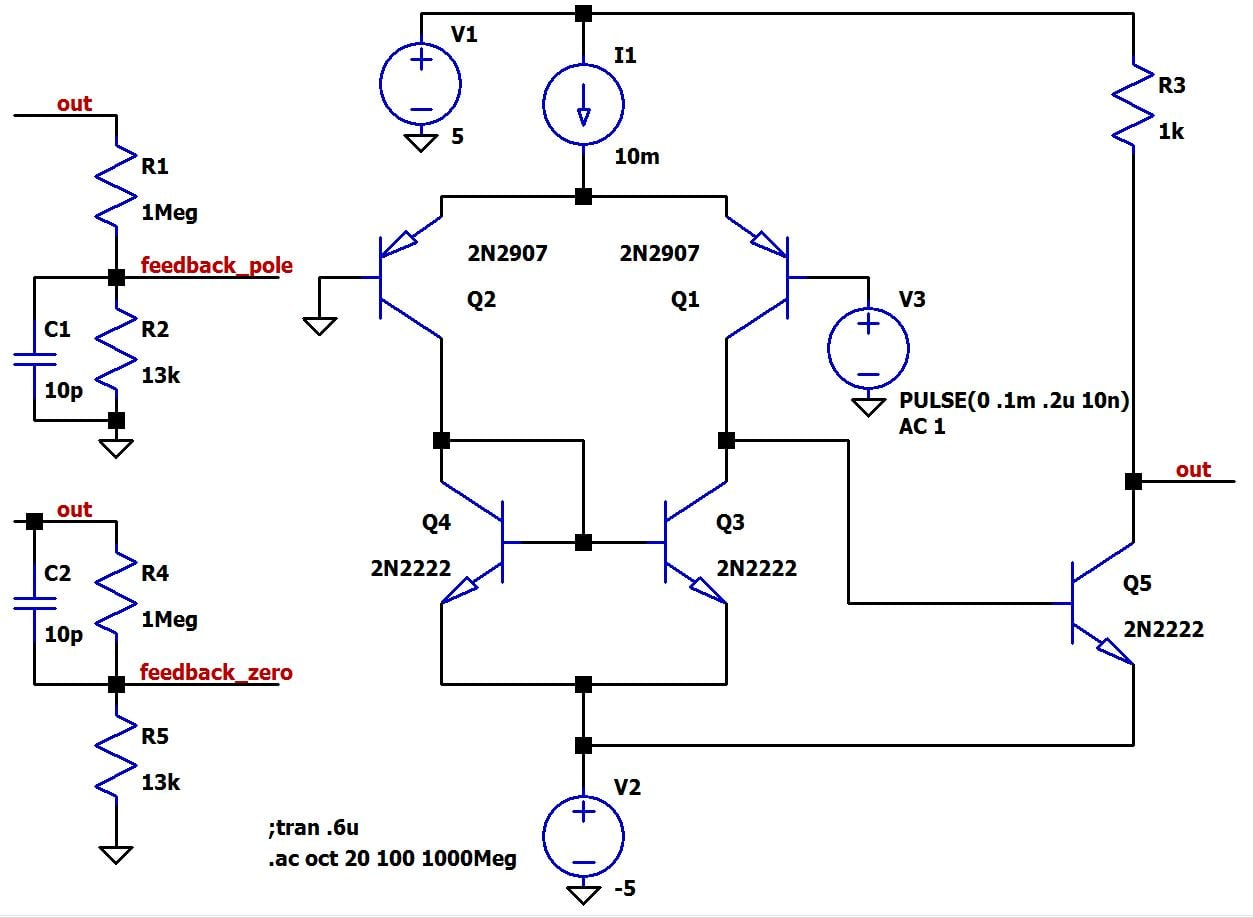Note that we now have two feedback networks—one has a capacitor added in parallel with the lower resistor, and the other has a capacitor in parallel with the upper resistor.

### Beware of Poles and Zeros

In previous articles we focused on feedback networks composed solely of resistors. You have to get up into the gigahertz range before a circuit is significantly affected by a resistor’s parasitic capacitance and inductance, so for our purposes we can assume that a resistor-only feedback network is independent of the frequency of the signal passing through. But now we need to look at what happens when the feedback factor β changes depending on signal frequency—in other words, now both A and β are functions of frequency, whereas previously A was a transfer function and β was a constant. Let’s start by looking at the A-and-1/β plot for the feedback network consisting of R1, R2, and C1.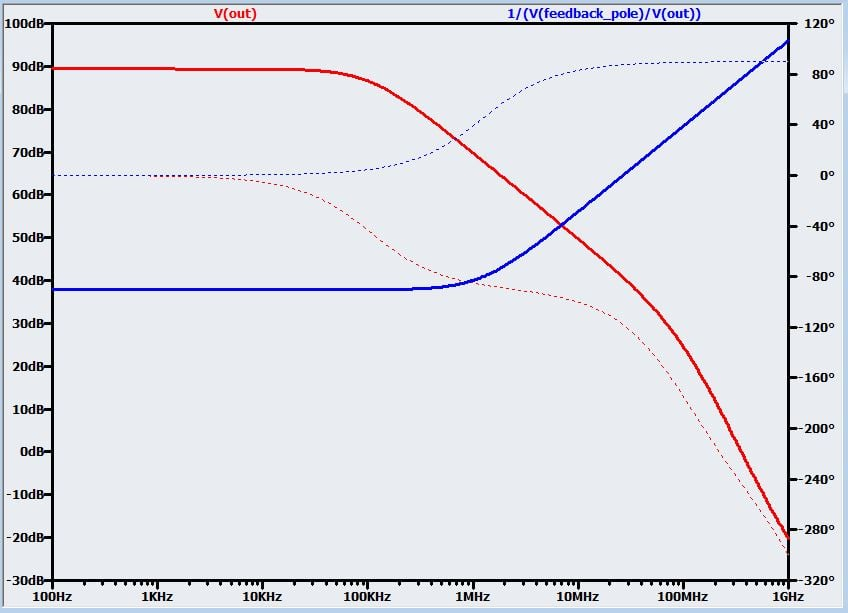As indicated in the legend, the blue line is the 20log(1/β) curve for the node labeled “feedback_pole.” This label is unavoidably confusing—“pole” implies decreasing magnitude response, whereas the curve exhibits increasing magnitude response. But “pole” refers to the fact that the feedback network itself includes a pole in the transfer function, meaning that the magnitude of β decreases as frequency increases past the pole frequency. The curve shown above exhibits the opposite response because it is a plot of 20log(1/β), not 20log(β). Likewise, the node labeled “feedback_zero” has a zero in the feedback transfer function, which corresponds to a 20log(1/β) curve with a decreasing magnitude response.

So first let’s recall our generalized intersection-point technique for analyzing stability: if the difference between the slope of the loop gain’s magnitude response and the slope of the feedback network’s magnitude response is not greater than 20 dB/decade at the point of intersection, the amplifier is sufficiently stable. Using this rule, we can readily see that our amplifier is no longer sufficiently stable. The addition of the capacitor in parallel with the lower feedback resistor causes the 20log(1/β) curve to increase by 20 dB/decade starting at around 1 MHz. Consequently, at the intersection point the slope of 20log(A) is -20 dB/decade and the slope of 20log(1/β) is +20 dB/decade. The difference in slopes is thus +20 dB/decade – (-20 dB/decade) = 40 dB/decade. This violates the generalized intersection-point criterion, so the circuit is not sufficiently stable.

### Exploring the Alternative Approach

This example aptly illustrates the advantages and intuitive aspects of this alternative approach to stability analysis:

• The visual nature of the two curves makes the essential information—i.e., is my circuit sufficiently stable—immediately apparent. If necessary, detailed information about the gain or phase margin can be obtained later by plotting the loop gain.
• The separate A and 1/β curves quickly convey helpful information about the factors influencing stability. In this case, it is obvious that the frequency response of the feedback network is the culprit, especially considering that by extending the low-frequency feedback response to the open-loop gain curve, we can confirm that the circuit would be sufficiently stable if the feedback network were frequency independent.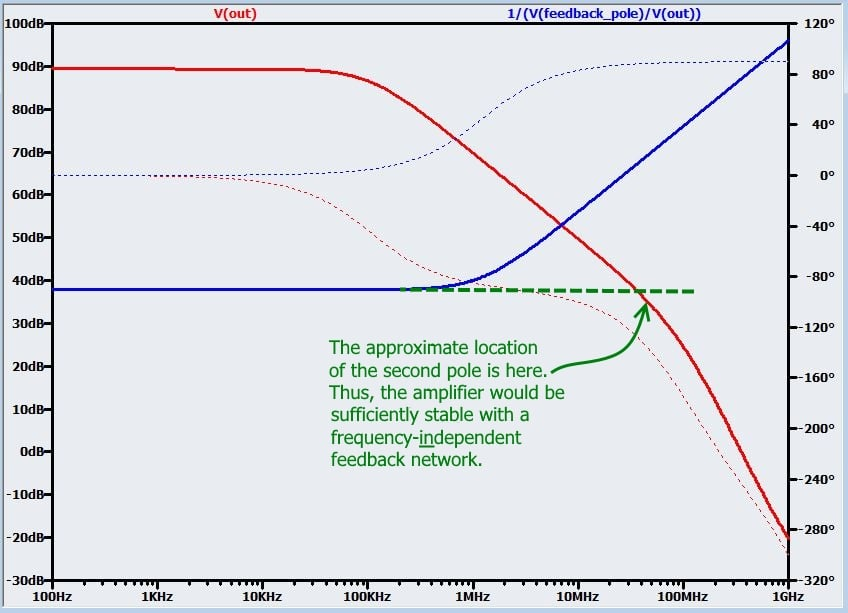• The distance between the feedback pole and the open-loop gain curve provides approximate though helpful information about how much we need to adjust the feedback frequency response—to make this circuit sufficiently stable, the 20log(1/β) curve must be flat (i.e., slope = 0) when it intersects the 20log(A) curve.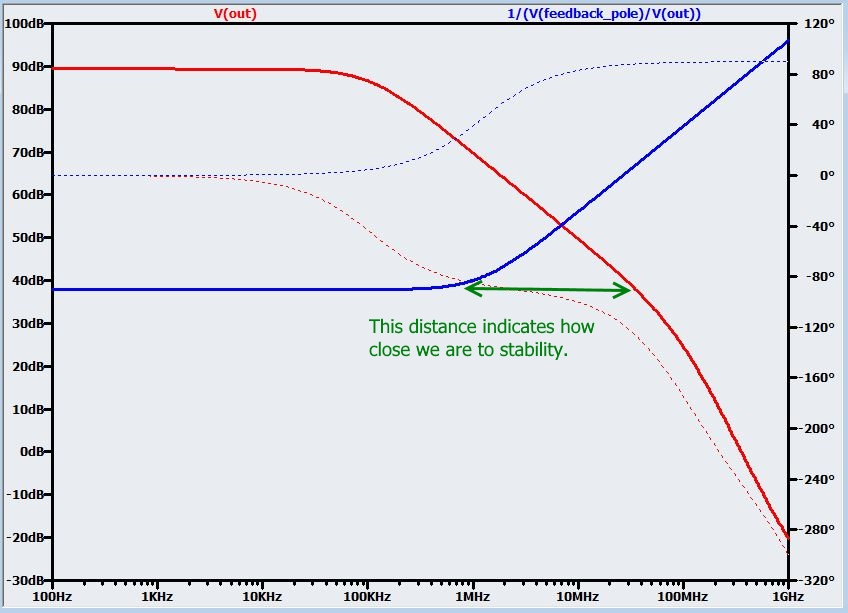• This method makes it clear that the frequency of the feedback pole must be higher than the intersection frequency, as demonstrated in the following plot, which includes curves for several feedback networks with different capacitance values. This plot also gives the corresponding phase margins (obtained via standard loop-gain analysis) for each curve, to give you an idea of the relationship between phase margin and the more qualitative stability information conveyed by the intersection of 20log(A) and 20log(1/β) curves.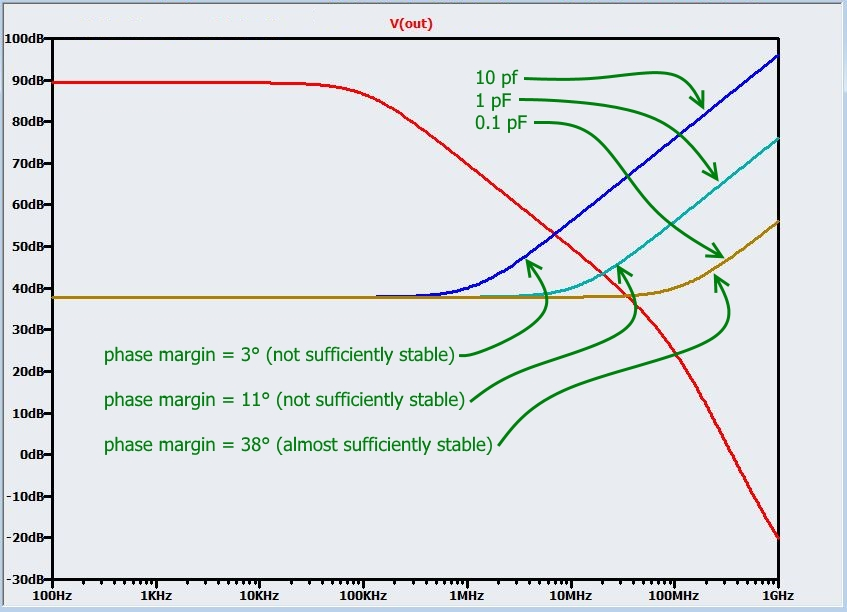• For this circuit and others like it, the A-and-1/β plot helps us to visualize something that might otherwise cause confusion. In previous articles we saw that higher closed-loop gain makes a negative-feedback amplifier more stable. But wait—we have a pole in the feedback network, leading to closed-loop gain that clearly increases with frequency, yet the circuit is less stable. What gives? The approximately 40 dB/decade difference in slopes tells us right away that we have stability problems; furthermore, the importance of slopes and pole locations in this analytical method helps us to remember that stability is fundamentally related to phase shift, which is caused by poles (and zeros). This is why higher closed-loop gain can lead to instability when β is a function of frequency: a pole in the feedback network also contributes additional phase shift, leading to more total loop-gain phase shift where loop-gain magnitude reaches unity.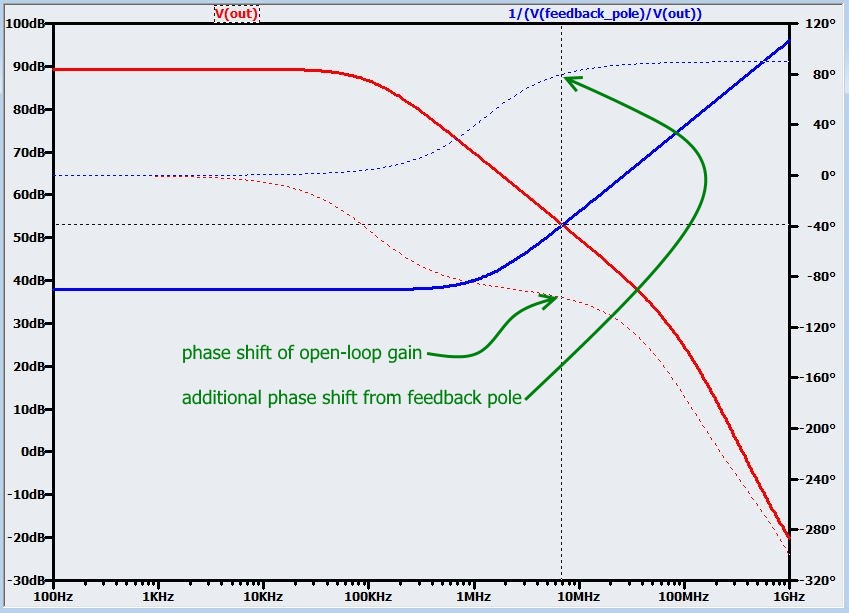### Zeros Can Cause Trouble Too

Here is the A-and-1/β plot for the feedback network consisting of R4, R5, and C2.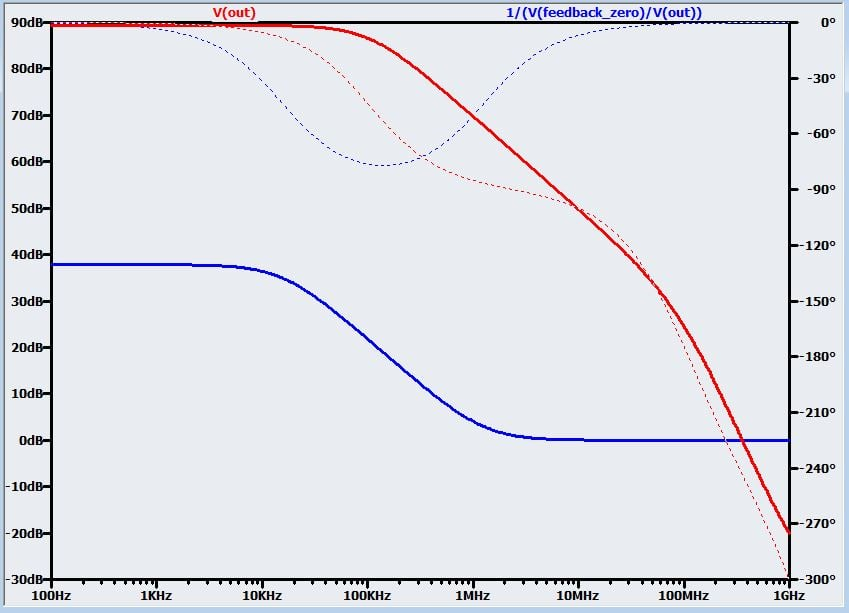As you can see, capacitance in the feedback network can be a lose-lose scenario. With the pole, additional phase shift robbed us of our stability. Now we have a zero, which has managed to move the intersection point to a 40 dB/decade section of the 20log(A) curve. The problem here is that we actually have a zero and a pole, a result you can understand by intuitively evaluating the feedback circuit: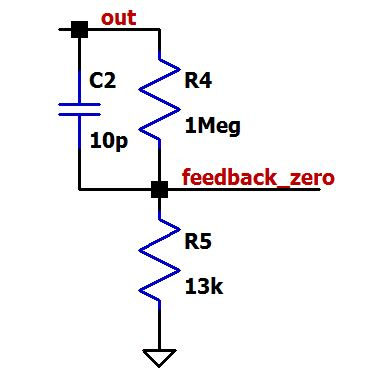As expected, the magnitude of β increases as the impedance of C2 decreases with increasing frequency. But then the curve levels out again because β approaches (and does not exceed) 1 as the impedance of C2 approaches zero. (A simple s-domain analysis will confirm that the transfer function has a root in the numerator and the denominator.)

The phase shift generated by the zero should help us, considering that its polarity is opposite to that of the problematic pole-generated phase shift that we saw in the first feedback network. But all the beneficial phase shift from the zero gets canceled out by the phase shift from the pole, such that the phase shift in the feedback network is almost zero at the intersection frequency. What we are left with is the decrease in closed-loop gain caused by the zero, and with this decreased closed-loop gain the 20log(1/β) trace intersects the 20log(A) trace at a frequency that is far beyond the second pole in the open-loop transfer function.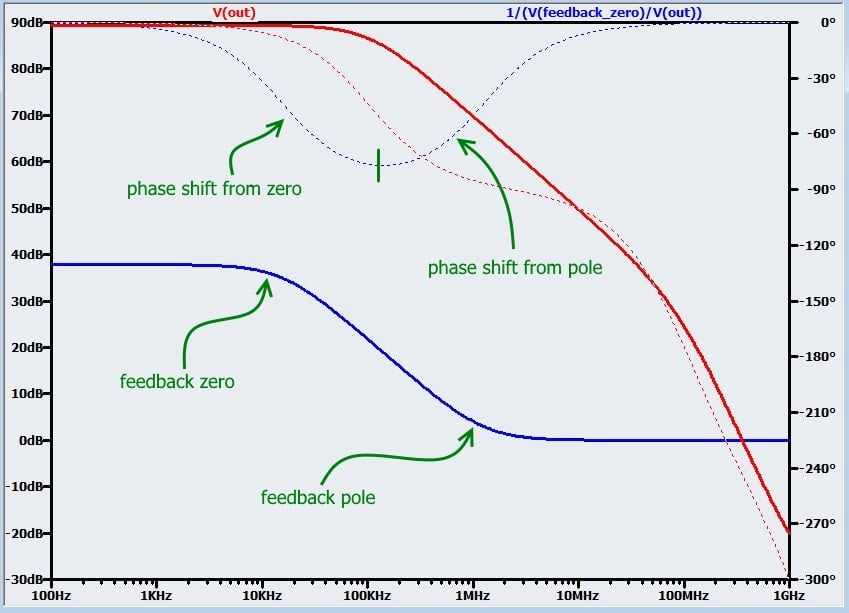### Conclusion

We have seen that frequency-dependent elements in a feedback network can cause all sorts of mischief for those who want an amplifier that amplifies rather than oscillates. Furthermore, you may have noticed that the capacitors used in the foregoing simulations were quite small. It is true that their baleful influence was exacerbated by the open-loop instability of this particular BJT amplifier and by the large resistors used in the feedback network; nonetheless, we should keep in mind that small amounts of added capacitance—in some cases perhaps even parasitic capacitance—can have a nontrivial effect on stability. In the next article we will use the concepts explored here to investigate the stability of an unusually oscillation-prone circuit: a photodiode transimpedance amplifier.

Next Article in Series: Negative Feedback, Part 8: Analyzing Transimpedance Amplifier Stability